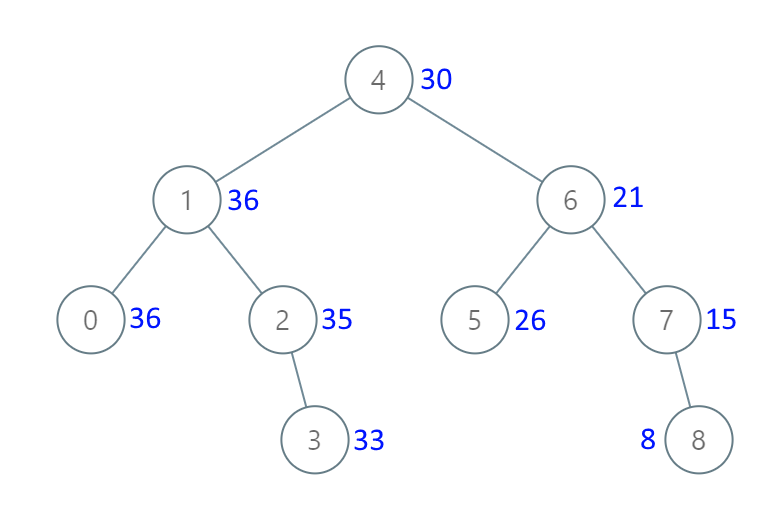1038.Binary Search Tree to Greater Sum Tree
1038.Binary Search Tree to Greater Sum Tree

Given the root of a binary search tree with distinct values, modify it so that every node has a new value equal to the sum of the values of the original tree that are greater than or equal to node.val.
As a reminder, a binary search tree is a tree that satisfies these constraints:
The left subtree of a node contains only nodes with keys less than the node's key. The right subtree of a node contains only nodes with keys greater than the node's key. Both the left and right subtrees must also be binary search trees.tree.png
1
Input: [4,1,6,0,2,5,7,null,null,null,3,null,null,null,8]
2
Output: [30,36,21,36,35,26,15,null,null,null,33,null,null,null,8]
Copied!
Note:
• The number of nodes in the tree is between 1 and 100.
• Each node will have value between 0 and 100.
• The given tree is a binary search tree.
If we want to get this greater sum tree, we should add the rightmost node, then add it's parent's node, and then, add it's parent's left child node. So we can set a sum to record the total sum from the rightmost node to the leftmose node. Code is as follows:
1
/**
2
* Definition for a binary tree node.
3
* struct TreeNode {
4
* int val;
5
* TreeNode *left;
6
* TreeNode *right;
7
* TreeNode(int x) : val(x), left(NULL), right(NULL) {}
8
* };
9
*/
10
class Solution {
11
int sum=0;
12
public:
13
TreeNode* bstToGst(TreeNode* root) {
14
if(!root ) return root;
15
if(!root->left && !root->right)
16
{
17
root->val +=sum;
18
sum=root->val;
19
return root;
20
}
21
if(root->right)
22
{
23
root->right = bstToGst(root->right);
24
25
}
26
root->val +=sum;
27
sum =root->val;
28
if(root->left)
29
{
30
root->left = bstToGst(root->left);
31
}
32
return root;
33
}
34
35
36
};
Copied!